# Universal side divisor

## Definition

A nonzero element$x$ in an commutative unital ring$R$ is termed a universal side divisor if$x$ satisfies the following two conditions:

•$x$ is not a unit.
• For any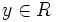$y \in R$, either$x$ divides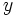$y$ or there exists a unit$u \in R$ such that$x$ divides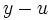$y - u$.

Equivalently, a non-zero non-unit element is a universal side divisor if and only if the unit balls centered around its multiples cover the whole ring.

### Equivalence up to associate classes

If$x,y$ are associate elements in a commutative unital ring$R$, then$x$ is a universal side divisor if and only if$y$ is a universal side divisor. For full proof, refer: Universal side divisor property is invariant upto associates

## Examples

• In the ring of rational integers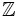$\mathbb{Z}$, the only universal side divisors are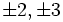$\pm 2, \pm 3$.$2$ is a universal side divisor because every integer is either a multiple of$2$ or differs by$1$ from a multiple of$2$.$3$ is a universal side divisor because every integer is either$0$,$1$, or$-1$ mod$3$. For any integer$n$ of absolute value greater than$3$, there is no way of subtracting a unit or zero from$2$ to get a multiple of$n$.
• In the polynomial ring over a field, the only universal side divisors are the nonconstant linear polynomials. For instance, in the ring$k[x]$,$x$ is a universal side divisor because for any polynomial, we can subtract the constant term of the polynomial to obtain a multiple of$x$, and the constant term is either zero or a unit. Similar reasoning applies for all the other nonconstant linear polynomials. On the other hand, for any polynomial of degree greater than$1$, there is no way of subtracting a unit from$x$ to get a multiple of that polynomial.